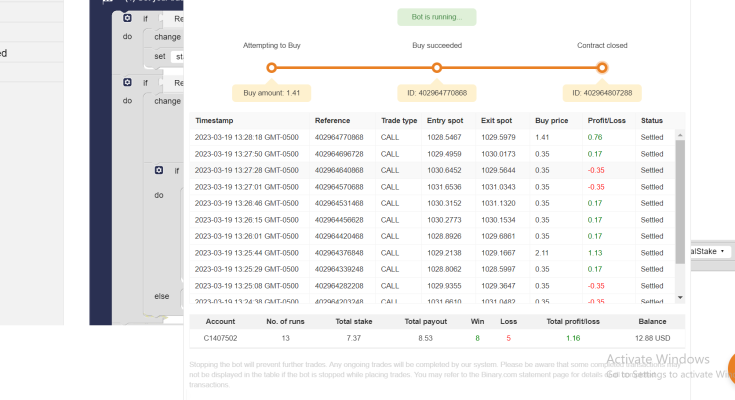### How this FREE Binary Bot works

This binary bot is designed to make a prediction about the last digit of the price for every movement of the price in a tick or second. The goal is to get the last digit of the price to be higher than 3.

If the last digit of the price is 0, 1, 2, or 3, the algorithm considers it to be a wrong prediction and implements the martingale strategy. On the other hand, if the last digit is 4, 5, 6, 7, 8, or 9, the algorithm considers it to be a correct prediction.

The initial stake for this strategy is 0.35c, and the reward is 0.20c if the prediction is correct. However, if the prediction is wrong, the algorithm implements the martingale strategy. In the martingale strategy, the stake is multiplied by a factor of 1.617, and the amount lost in the previous bet is added to the new stake.

For example, if the first bet is wrong and the stake is 0.35c, the new stake for the second bet would be 0.35 * 1.617 + 0.35 = \$0.92. If the second bet is also wrong, the new stake for the third bet would be 0.92 * 1.617 + 0.92 = \$2.40. This process continues until the algorithm makes a correct prediction.

The martingale strategy can be risky as it increases the stake exponentially after each loss. Therefore, it’s important to use this strategy cautiously and not invest more than you can afford to lose.

### Recommendation for Small Deposit Binary BOT

• Work best between 7 AM to 1 AM GMT time.
• Important
• Run Chrome in Incognito mode to avoid tracking by the Deriv platform.
• Avoid running the bot for an extended period during a trading session(max 1 Hour)